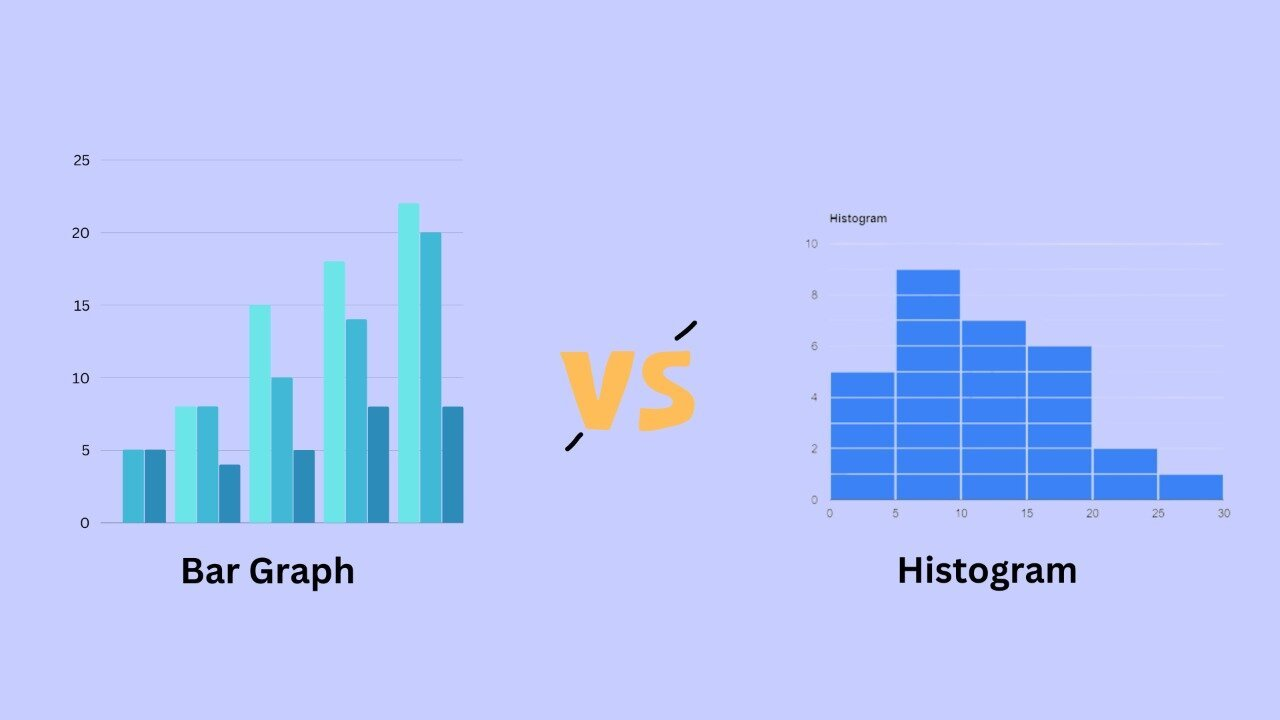# What is the difference between the Bar graph and a Histogram?A bar graph and a histogram are two common types of data graphical representations. While they may appear identical, there are several key differences between the two that must be understood.

A bar graph is a chart that employs bars to depict the frequency or quantity of various types of data. The bars can be vertical or horizontal, and they are usually positioned horizontally or vertically to make it easier to compare the different groups. Bar graphs are useful for depicting data that can be broken down into discrete groups, such as the number of pupils in each grade level at a school.

In contrast, a histogram is a graph that depicts the distribution of numerical data. It is a form of bar chart that displays the frequency or quantity of observations within distinct numerical ranges known as bins. Bins are often stated as non-overlapping, sequential intervals of a variable. The histogram shows the amount of observations that fall into each bin and offers a visual picture of the data distribution. This can be beneficial for spotting patterns and trends in data as well as establishing comparisons between different datasets.

One of the primary distinctions between a bar graph and a histogram is the type of data they represent. A bar graph is used to depict categorical data, whereas a histogram is used to depict continuous numerical data. That is, a bar graph is used to compare several categories, but a histogram is used to depict the distribution of a single variable.

Another distinction between a bar graph and a histogram is how the data is organised. A bar graph typically arranges the categories along a horizontal or vertical axis, with the bars showing the frequency or quantity of each category. The bins of a histogram are placed along the horizontal axis, with the vertical axis denoting the frequency or number of observations within each bin.

Another significant distinction between a bar graph and a histogram is how the bars are sized. The bars in a bar graph can be any size, and they are usually scaled to make it easy to compare the different data points. categories. The bars in a histogram are scaled such that they have the same height. the same width, allowing for accurate comparisons between the separate bins.

To summarise, while a bar graph and a histogram may appear similar, there are several significant distinctions between the two. A bar graph is used to depict categorical data, whereas a histogram is used to depict continuous numerical data. A bar graph is used to compare several categories, whereas a histogram is used to depict the distribution of a single variable. A bar graph employs bars of varied sizes, whereas a histogram employs bars of equal width. Understanding these distinctions might assist you in selecting the best type of graph for your data.